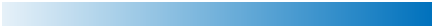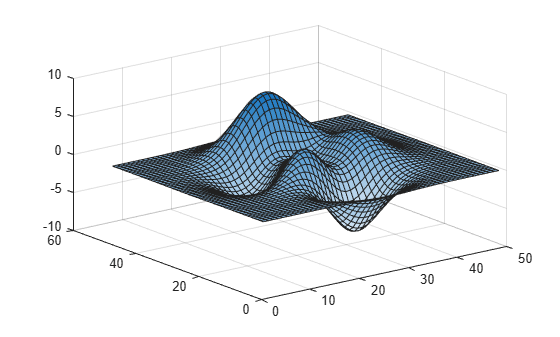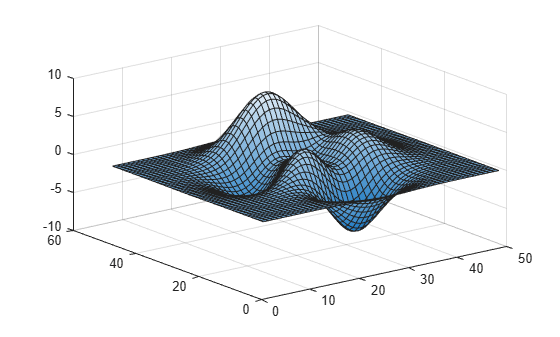# sky

Sky colormap array

Since R2023a

•## Syntax

``c = sky``
``c = sky(m)``

## Description

example

````c = sky` returns the sky colormap as a three-column array with the same number of rows as the colormap for the current figure (`gcf`). If no figure exists, the number of rows is 256. Each row in the array contains the red, green, and blue intensities for a specific color. The intensities are in the range [0, 1], and the color scheme looks like this image.```

example

````c = sky(m)` returns the colormap with `m` colors.```

## Examples

collapse all

Plot a surface and assign the sky colormap.

```surf(peaks) colormap("sky")```Get the sky colormap array and reverse the order. Then apply the modified colormap to the surface.

```c = sky; c = flipud(c); colormap(c)```Get a downsampled version of the sky colormap containing only twenty colors. Then display the contours of the peaks function by applying the colormap and interpolated shading.

```c = sky(20); surf(peaks) colormap(c) shading interp```Heatmap charts use the 256-sampling of the `sky` colormap by default. You can change the sampling by specifying the `Colormap` name-value argument.

For example, create two heatmap charts in a 2-by-1 tiled chart layout. The top chart uses the default set of 256 colors, and the bottom chart uses only two colors.

```tiledlayout(2,1,TileSpacing="compact") % Top chart with default colors nexttile heatmap(magic(10)) % Bottom chart with two colors nexttile heatmap(magic(10),Colormap=sky(2))```## Input Arguments

collapse all

Number of colors, specified as a nonnegative integer. The default value of `m` is the number of colors in the current figure's colormap. If no figure exists, the default value is `256`.

Data Types: `single` | `double`

## Version History

Introduced in R2023a